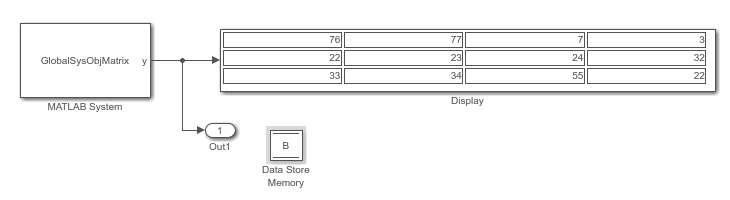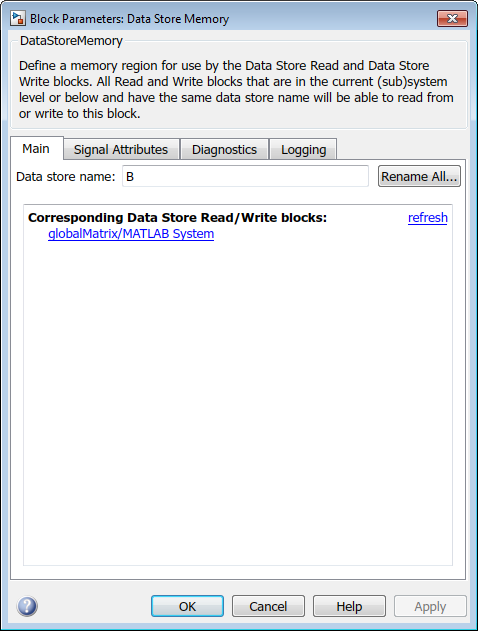## Use Global Variables in System Objects

Global variables are variables that you can access in other MATLAB® functions or Simulink® blocks.

### System Object Global Variables in MATLAB

For System objects that are used only in MATLAB, you define global variables in System object™ class definition files in the same way that you define global variables in other MATLAB code (see Global Variables).

### System Object Global Variables in Simulink

For System objects that are used in the MATLAB System block in Simulink, you also define global variables as you do in MATLAB. However, to use global variables in Simulink, you must declare global variables in the `stepImpl`, `updateImpl`, or `outputImpl` method if you have declared them in methods called by `stepImpl`, `updateImpl`, or `outputImpl`, respectively.

You set up and use global variables for the MATLAB System block in the same way as you do for the MATLAB Function block (see Data Stores (Simulink) and Share Data Globally (Simulink)). Like the MATLAB Function block, you must also use variable name matching with a Data Store Memory block to use global variables in Simulink.

For example, this class definition file defines a System object that increments the first row of a matrix by 1 at each time step. You must include `getGlobalNamesImpl` if the class file is P-coded.

```classdef GlobalSysObjMatrix < matlab.System methods (Access = protected) function y = stepImpl(obj) global B; B(1,:) = B(1,:)+1; y = B; end % Include getGlobalNamesImpl only if the class file is P-coded. function globalNames = getGlobalNamesImpl(~) globalNames = {"B"}; end end end ```
This model includes the `GlobalSysObjMatrix` object in a MATLAB System block and the associated Data Store Memory block.